# Help with Example Electrostatics Model

Hello, I am trying to model a simple 3D electrostatics problem. A metallic object at 1 Volt is surrounded by air and is positioned above a ground plane. The upper part of the dome is a “distant surface” air boundary. My objective is to obtain a result containing the surface charge density at each mesh node on the surface of the energized object. A .step CAD file is attached (dome.step). Since I don’t care about anything internal to the cuboid, the volume consists of a dome with a cuboid void.
https://ngsolve.org/media/kunena/attachments/1333/dome.zip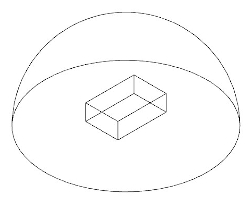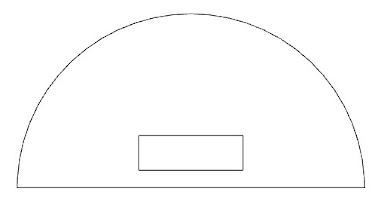I normally use the netgen gui to mesh and export to Elmer Format. However, I learned that this could be done in ngsolve with scripting.

I have been able to get as far importing the .step file and meshing the object (code below). The imported step file has surfaces: 1 = air boundary, 2 = ground plane, 3 through 8 = cuboid.

Could someone point me in the right direction or give example scripts that show:
[ol]
[li] How to name boundaries (I would like to set the air boundary to bc 1, ground plane to bc 2, and energized surfaces to bc 3.[/li]
[li] How to assign potentials to a bc [/li]
[li] How to assign a Nuemann boundary condition to the air boundary[/li]
[li] How to solve and get an output with the charge density at the surface nodes of the energized cuboid [/li]
[/ol]

I’m really stuck on the first item. I might be able to find the other three if I can get that one figured out, but if the others are quick for someone to point out, I would really appreciate the assistance. Thanks!
.
.

``````from netgen.occ import *

geo = OCCGeometry('C:/Temp/dome.step') #import step file

for x in range(2,8):  #iterate through surfaces of cuboid
geo.SetFaceMeshsize(x,25)  #set mesh size for cuboid surfaces

geo.SetFaceMeshsize(0,100)  #set mesh size for air boundary
geo.SetFaceMeshsize(1,25)  #set mesh size for ground plane
mesh = geo.GenerateMesh(maxh = 100,grading = 0.75)  #mesh the volume with prescribed size and grading

mesh.Save('C:/Temp/dome.vol') #save mesh out``````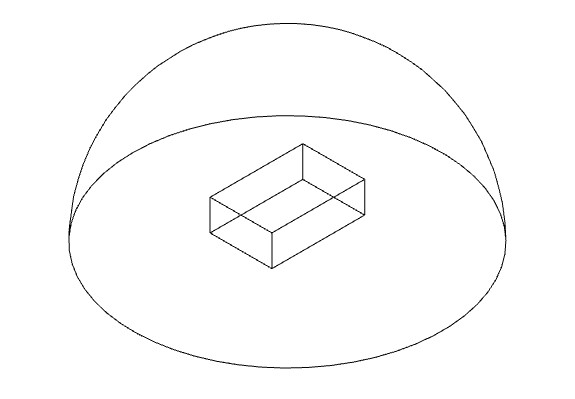Attachment: domeisometricview.jpg

Attachment: mesh.jpg

Attachment: domesideview.jpg

Attachment: dome.zip

Hi jleman,

you can set materials (1 based index) by

``mesh.SetMaterial(int, string)``

and boundary conditions by

``mesh.SetBCName(int, string)``

For setting non-homogeneous Dirichlet boundary conditions I recommend this tutorial and this.

Neumann boundary conditions should be straight-forward with 2). For homogeneous Neumann conditions you mostly have the “do-nothing” conditions, otherwise it goes naturally into the right-hand side of your problem.

The tutorials in 2) should be a good starting point here.

Best,
Michael

[ol]
[li]Per your suggestion I ran the code with the SetBCName function applied as shown below. The code runs fine (no errors), but when I explore the mesh boundary conditions in the netgen gui, all boundaries have the name ‘voltage’ (no ‘air’ and ‘ground’ boundaries). Face index 1 and 2 should be ‘air’ and ‘ground’ respectively. Suggestions?[/li]
[li]Also, how do I assign a face index to a bc property? In this case I need face index 1 to be bc property 1, face index 2 to be bc property 2, and face indices 3 through 8 to be bc property 3.[/li]
[/ol]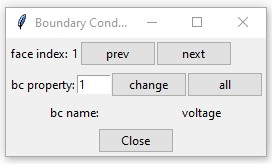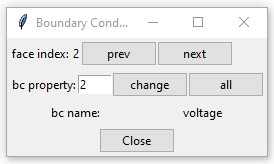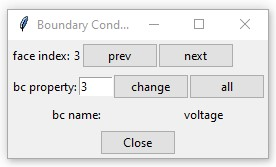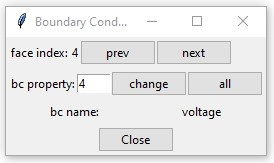etc…

``````from netgen.occ import *
from ngsolve import *

geo = OCCGeometry('C:/00_Temp/netgen testing/dome.step')

for x in range(2,8):
geo.SetFaceMeshsize(x,100)

geo.SetFaceMeshsize(0,1000)
geo.SetFaceMeshsize(1,250)
mesh = geo.GenerateMesh(maxh = 1000,grading = 0.75)

mesh.SetBCName(0,'air')
mesh.SetBCName(1,'ground')

for x in range(2,8):
mesh.SetBCName(x,'voltage')

mesh.Save('C:/00_Temp/netgen testing/dome.vol')
``````

Attachment: img1.jpg

Attachment: img2.jpg

Attachment: img3.jpg

Attachment: img4.jpg

Hi jleman,

with the attached code I get also air and ground as boundaries
Output:
boundaries = (‘air’, ‘ground’, ‘voltage’, ‘voltage’, ‘voltage’, ‘voltage’, ‘voltage’, ‘voltage’)

Best
Michael

https://ngsolve.org/media/kunena/attachments/889/dome.py

Attachment: dome.py

Is there a way to get the following information from the electrostatic model once it has solved?

1. x,y,z coordinates of all nodes
2. surface charge density calculated for surface node of the energized block

I noticed that the .vol mesh file saved after naming the boundaries does not seem to properly retain the data. When I open the .vol file manually with the netgen gui then go to mesh=>edit boundary conditions, all the boundary names say ‘voltage’. If I move the `ngmesh.SetBCName(1,'ground')` line so that it is the last of the boundary naming entries then all boundaries show up as “ground”. Is this a bug?

Ultimately I am trying to export the mesh to an elmerfem format then user elmer to solve the electrostatics problem and get a result that contains all the node x,y,z locations and surface density values at each node. Perhaps it would be easier to do all that from within ngsolve?

Hi jleman,

if I load the saved mesh in python via

``mesh = Mesh('dome.vol')``

all boundaries seem to be saved and loaded properly.

With

``````for v in mesh.vertices:
print(v.point)``````

you get all mesh points.

If you solve the problem in NGSolve and assume your solution is saved in the GridFunction gfu then you can access data at a point p=(p_x, p_y, p_z) by

``value = gfu(mesh(p_x,p_y,p_z))``

If you have a CoefficientFunction Q representing the charge density you can get values on the surface by

``value = Q(mesh(p_x,p_y,p_z, BND))``

Of course the point must be a surface point on the mesh.

If you have the equations by hand I would recommend doing everything in NGSolve via Python.

Best
Michael

Hello mneunteufel,

I was wondering if you still have access to this dome.py file or you remember how to do this, as it would be very helpful for my current project. Thanks!

Hi,
This code snippet should solve this problem:

``````from netgen.occ import *
from ngsolve import *
air = Sphere((0,0,0), 1)
air.faces.name = "air"

ground = HalfSpace((0,0,0), (0,0,-1))
ground.faces.name = "ground"

box = Box((-0.1,-0.1,-0.1), (0.1,0.1,0.1))
box.faces.name = "volt"
box.faces.maxh = 0.02

domain = (air * ground) - box

geo = OCCGeometry(domain)

mesh = Mesh(geo.GenerateMesh(maxh=0.2))
mesh.Curve(3)
Draw(mesh)

fes = H1(mesh, order=3, dirichlet="ground|volt")
u,v = fes.TnT()

eps = 1

a = BilinearForm(fes)

u = GridFunction(fes)